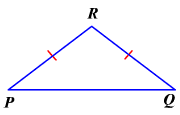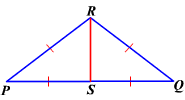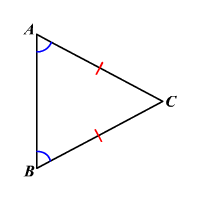# Isosceles Triangle Theorem

If two sides of a triangle are congruent , then the angles opposite to these sides are congruent.$\angle P\cong \angle Q$

Proof:

Let $S$ be the midpoint of $\stackrel{¯}{PQ}$ .

Join $R$ and $S$ .Since $S$ is the midpoint of  $\stackrel{¯}{PQ}$ , $\stackrel{¯}{PS}\cong \stackrel{¯}{QS}$ .

$\stackrel{¯}{RS}\cong \stackrel{¯}{RS}$

It is given that $\stackrel{¯}{PR}\cong \stackrel{¯}{RQ}$

Therefore, by SSS ,

$\Delta PRS\cong \Delta QRS$

Since corresponding parts of congruent triangles are congruent,

$\angle P\cong \angle Q$

The converse of the Isosceles Triangle Theorem is also true.

If two angles of a triangle are congruent, then the sides opposite those angles are congruent.If $\angle A\cong \angle B$ , then $\stackrel{¯}{AC}\cong \stackrel{¯}{BC}$ .